# Real Numbers Class 10 Extra Questions Maths Chapter 1 with Solutions Answers

Here you will find Real Numbers Class 10 Extra Questions Maths Chapter 1 with Answers Solutions, Extra Questions for Class 10 Maths are solved by experts and will guide students in the right direction.

## Extra Questions for Class 10 Maths Real Numbers with Answers Solutions

Extra Questions for Class 10 Maths Chapter 1 Real Numbers with Solutions Answers

### Real Numbers Class 10 Extra Questions Objective Type

Question 1.
The graph of y = f(x) is given. The no. of zeros of f(x) will be: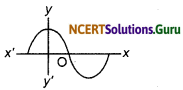(a) 1
(b) 3
(c) 2
(d) 4
(b) 3
Solution.
As graph of/(x) cut the x and at 3 points
∴ Zeros of fix) are 3.
Choice (b) is correct.

Question 2.
For some integer m, the form of every positive even integer will be:
(a) m
(b) m + 1
(c) 2m
(d) 2m + 1
(c) 2m
Solution.
2m is even integer
Hence, Choice (c) is correct.Question 3.
The rational number which can be expressed as a terminating decimal number will be:
(a) $$\frac {77}{210}$$
(b)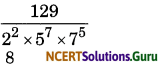(c) $$\frac {13}{3125}$$
(d) $$\frac {8}{17}$$
(c) $$\frac {13}{3125}$$
Solution.
As the prime factorization of denominators of $$\frac {13}{3125}$$ is only at the ninth place of 2 and 5.
Hence, choice (c) is correct.

Question 4.
For some integer n, the form of every positive odd integer will be:
(a) n
(b) n + 1
(c) 2n
(d) 2n + 1
(d) 2n + 1
Solution.
For some integer n, the odd positive integer will be (2n + 1)
Hence, choice (d) is correct.

Question 5.
Which of the following graph is not of a quadratic polynomial?
(a)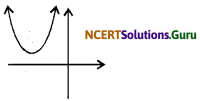(b)(c)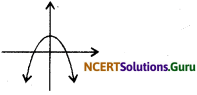(d)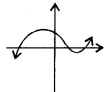(d)Solution.
Choice (d) is correct, (d)Question 6.
Decimal expansion of rational number $$\frac {14587}{1250}$$ will end after the following decimal places:
(a) one
(b) two
(c) three
(d) four
(d) four
Solution.
Given Rational Number $$\frac {14587}{1250}$$
The factors of the denominator 1250 is 5 × 2
So the Number is terminating decimal expansion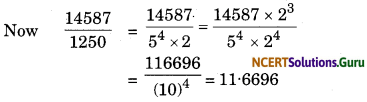∴ Number will end after 4 is decimal places.
Hence choice (d) is correct. Answer:

Question 7.
The decimal expansion of $$\frac {131}{120}$$ will terminate after how many places of decimals?
(a) 3
(b) 4
(c) 1
(d) 2
(a) 3Question 8.
The decimal expansion of the rational number $$\frac{11}{2^{3} \cdot 5^{2}}$$ will terminate after:
(a) one decimal place
(b) two decimal places
(c) three decimal places
(d) more than 3 decimal places.
(c) three decimal places

Question 9.
The decimal expansion of the rational number $$\frac{43}{2^{4} \times 5^{3}}$$will terminate after:
(a) 3 places
(b) 4 places
(c) 5 places
(d) 1 place.
(b) 4 places

Question 10.
The decimal expansion of the rational numbers $$\frac{23}{2^{2} \cdot 5}$$ will terminate after:
(a) one decimal place
(b) two decimal places
(c) three decimal places
(d) more than three decimal places.
(b) two decimal places

### Real Numbers Class 10 Extra Questions Very Short Answer Type

Question 1.
Use Euclid’s division algorithm to find the HCF of:
(i) 135 and 225
(ii) 196 and 38220
(iii) 867 and 255
Solution:
By Euclid’s division algorithm, we have Dividend = (Divisor × Quotient + Remainder)
225 = 135 × 1 + 90We apply Euclid’s division algorithm on divisor 135 and the remainder 90.
Dividend = (Divisor × Quotient + Remainder)
135 = 90 × 1 + 45 1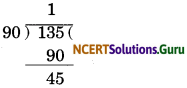Again, we apply Euclid’s division algorithm on divisor 90 and remainder 45.
90 = 45 × 2 + 0
∴ HCF (135,225) =45

(ii) We have,
Dividend = 38220 and Divisor = 196
Dividend = (Divisor × Quotient + Remainder)
38220 = 196 × 195 + 0
Hence, HCF (196, 38220) = 196

(iii) By Euclid’s division algorithm,
we have
Dividend = (Divisor × Quotient + Remainder)
867 = 255 + 3 + 102
We apply Euclid’s division algorithm on the divisor 255 and the remainder 102.
Dividend = (Divisor × Quotient + Remainder)
255 = 102 × 2 + 51
Again, we apply Euclid’s division algorithm on the divisor 102 and the remainder 51.
Dividend = (Divisor × Quotient + Remainder)
102 = 51 × 2 + 0
∴ HCF (867, 255) = 51Question 2.
Using Euclid’s division algorithm prove that: 847, 2160 are co-primes/relatively prime.
Solution.
Definition of co-primes or relatively primes : Two numbers are said to be co-prime or relatively prime. If their HCF is 1. Hence to prove 847 and 2160 as co-prime numbers we will find their HCF and which should be 1.
Now steps to find HCF will be as under
2160 = 847 × 2 + 466
847 = 466 × 1 + 381
466 = 381 × 1 + 85
381 = 85 × 4 + 41
85 = 41 × 2 + 3
41 = 3 × 13 + 2
3 = 2 × 1 + 1 .
2 = 1 × 2 + 0
Therefore, the HCF = 1.
Hence, the numbers are co-prime relatively prime.

Question 3.
Find the largest number that divides 628, 3129 and 15630 to leave remainders 3, 4 and 5 respectively.
Solution.
Required number = HCF of (628 – 3), (3129 – 4) and (15630 – 6) i.e., required number
= HCF of 625, 3125,15625
To find HCF of 625, 3125 and 15625 first we will find HCF of 625 and 3125 and then we will find HCF of HCF of (625 & 3125) and 15625.
Step (1): HCF of 625 and 3125 by using Euclid’s division algorithm is
3125 = 625 × 5 + 0
⇒ HCF of 625 and 3125 is 625.
Step (2): Now HCF of625 and 15625 will be : 15625 = 625 × 25 + 0
⇒ HCF of 625 and 15625 is 625.
Hence HCF of 625, 3125 and 15625 is 625.
Hence required number is 625.Question 4.
An Army contingent of 616 members is to march behind an army bond of 32 members in a parade. The two groups are to march in the same number of columns. What is the maximum number of columns in which they can march?
Solution.
To find the maximum number of columns, we have to find the HCF of 616 and 32. By Euclid’s division algorithm 19 4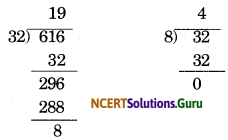i.e., 616 = 32 × 19 + 8 i.e., 32 – 8 × 4 + 0
∴ The HCF of 616 and 32 is 8.
Hence, maximum number of columns is 8.

Question 5.
Show that the square of an odd positive integer is of the form 8m + 1, for some whole number m.
Solution.
Any positive odd integer is of the form 2q + 1, where q is a whole number.
Therefore,
(2q + 12 = 4q2 + 4q + 1
= 4 q(q + 1) + 1 …(1)
q(Question + 1) is either 0 or even because out of q and q + 1 one of the number is even. So, it is 2m, where m is a whole number.
Therefore, (2q + 1)2
= 4.2 m + 1 = 8m + 1. [From (1)]Question 6.
Given that HCF (306, 657) = 9, find LCM (306, 657).
Solution.
We have,
HCF (306, 657) = 9
We know that,
Product of LCM and HCF
= Product of two numbers
⇒ LCM × 9 = 306 × 657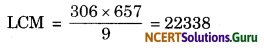Hence, LCM (306, 657) = 22338

### Real Numbers Class 10 Extra Questions Short Answer Type

Question 1.
Use Euclid’s division lemma to show that the square of any positive integer is either of the form 3m or 3m + 1 for some integer m.
Solution:
By Euclid’s division algorithm, we have
a – bq + r …(i)
On putting b = 3 in Eq (i), we get a = 3q + r, [0 ≤ r < 3, i.e. , r = 0, 1, 2]
If r = 0 ⇒ a – 3q
⇒ a2 = 9q2 …(ii)
If r = 1 ⇒ a = 3q + 1
⇒ a2 = 9q2 + 6q + 1 …(iii)
If r = 2 ⇒ a = 3q + 2
⇒ a2 = 9q2 + 12g + 4 …(iv)
From Eq (ii), 9q2 is a square of the form 3m, where m 3q2
From Eq (iii), 9q2 + 6q + 1 i.e., 3(3q2 + 2q) + 1 is a square which is of the form
3m + 1, where m = 3q2 + 2q
From Eq (iv) 9q2 + 12q + 4 i.e.,3(3q2 + 4q + 1) + 1 is a square which is of the form 3m + 1, where m – 3q2 + 4q + 1.
∴ The square of any positive integer is either of the form 3m or 3m + 1 for some integer m.Question 2.
Show that that square of an odd positive integer can be of the form 6q + 1 or 6q + 3 for some integer q.
Solution:
We know that any positive integer can be of the form 6m, 6m + 1, 6m + 2, 6m + 3, 6m + 4 or 6m + 5, for some integer m.
Thus, an odd positive integer can be of the form 6m + 1, 6m + 3, or 6m + 5.
Thus we have :
(6m + 1)2 – 36m2 + 12m + 1
= 6(6m2 + 2m) + 1 = 6q + 1,
q = 6m2 + 2m is an integer.
(6m + 3)2 – 36m2 + 36m + 9
6(6m2 + 6m + 1) + 3
= 6q + 3,
q = 6m2 + 6m + 1 is an integer.
(6m + 5)2 36m2 + 60m + 25
= 6(6m2 + 10m + 4) + 1 = 6q+ 1, q
= 6m2 + 10m + 4 is an integer.
Thus, the square of an odd positive integer can be of the form 6q + 1 or 6q + 3.

Question 3.
Find the LCM and HCF of the fo11owing pairs of integers and verify that LCM × HCF = product of the two numbers.
(i) 26 and 91 (ii) 510 and 92
Sol:
(i) 26 and 91
26 = 2 × 13 and 91 = 7 × 13
LCM of 26 and 91
= 2 × 7 × 13 = 182
and HCF of 26 and 91 = 13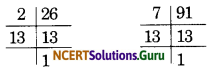Now, 182 × 13 = 2366 and 26 × 91 = 2366
Hence, 182 × 13 = 26 × 91

(ii) 510 and 92
510 = 2 × 3 × 5 × 17
and 92 = 2 × 2 × 23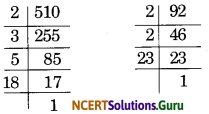∴ LCM of 510 and 92
= 2 × 2 × 3 × 5 × 17 × 23 = 23460
and HCF of 510 and 92 = 2
Now, 23460 × 2 = 46920
and 510 × 92 = 46920
Hence, 23460 × 2 = 510 × 92Question 4.
Prove that 5 + √3 is an irrational number.
Solution:
Let us assume, to the contrary, that 5 + √3 is rational.
So that we can find integers p and q(q ≠ 0) such that
5 + √3 = P/q,
Where p and q are coprime.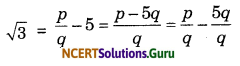Since, p and q are integers, we get $$\frac {p}{q}$$ – $$\frac {5q}{q}$$ is a rational. So √3 is rational.
But this contradict the fact that √3 is irrational.
So, we conc1ude that 5 + √3 is irrational.
Proved

Question 5.
With out performing 1ong division, show whether the rational number is terminating decimal or non-terminating decimal. Find a1so its decimal expansion without performing actual division.
Solution:
Given Number is $$\frac {17}{8}$$ in this 17 and 8 are coprime and the denominator is on1y the mu1tip1e of 2.
∴ $$\frac {17}{8}$$ is a terminating decimal.
Now,Question 6.
Without performing 1ong division procidure, show that the rational Number $$\frac {17}{90}$$ is terminating decimal or non terminating repeating decimal.
Solution:
Given, Number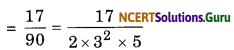In denominator it contains 32 therefore this number is non-terminating repeating decimal.

Question 7.
Exp1ain, Why
(17 × 5 × 11 × 3 × 2 + 2 × 11) is a composite number.
Solution:
17 × 5 × 11 × 3 × 2 + 2 × 11
= 2 × 11 [17 × 5 × 3 + 1]
= 22 × 256 = 2 × 11 × 4 × 4 × 4 × 4
Which is a product of more than two prime numbers i.e., 2 and 11. Hence it is a composite number.

Question 8.
Without performing the 1ong division process, find whether the rational Number $$\frac {987}{10500}$$ is terminating or non terminating decimal. Give reason of your answer.
Solution: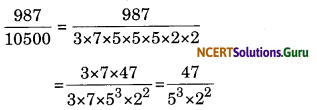∵ The prime factor of denominator is of the form 53 × 22
∴ The given rational number is terminating decimal.### Real Numbers Class 10 Extra Questions Long Answer Type

Question 1.
Prove that √2 is irrational.
Solution:
If possible, let √2 be rational and let its simp1est form be $$\frac {a}{b}$$ .
Then, a and b are integers having no common factor other than 1, and b ≠ 0.
Now,[on squaring both sides]
⇒ 2 b2 = a2 …(i)
⇒ 2 divides a2 [∵ 2 divides 2b2]
⇒ 2 divides a
[ ∵ 2 is prime and divides c2 ⇒ 2 divides a]
Let a = 2c for some integer c.
Putting a = 2c in (i), we get
2b2 = 4c2
⇒ b2 = 2c2
⇒ 2 divides b2 [ ∵ 2 divides 2c2]
⇒ 2 divides b[ ∵ 2 is prime and 2 divides b2 ⇒ 2 divides b]
Thus, 2 is a common factor of a and b.
But, this contradicts the fact that a and b have no common factor other than 1.
The contradiction arises by assuming that √2 is rational.
Hence, √2 is irrational.Question 2.
Why n is an irrational number.
Solution:
We know that the ratio of circumference of a circle to 1ength of its corresponding diameter is known as ‘π’ (pie).
i.e., π = $$\frac {c}{d}$$
Now, read this to understand why ‘π’ is an irrational number.
If we take circumference of a circle as an integer (natural number) then we find length of its corresponding diameter comes in decimal not an integer (natura1 number)] so ratio of $$\frac {c}{d}$$ is not in the form of $$\frac {p}{q}$$, i.e., p and q bothare not integers.

Simi1arly if we take diameter of circle as an integer (natura1 number) then circumference of its corresponding circle comes out to be in the decimal form so again ratio $$\frac {c}{d}$$ = π does not comes in the form of $$\frac {p}{q}$$ because diameter, i.e., ‘q’ is an integer and ‘p’ is in decimal form.

So it is clear that for any circle if length of diameter is natura1 number then circumference is the decimal form or if circumference is a natura1 number then diameter is a decimal number. So we can say that the ratio $$\frac{\pi}{d}$$ can never come in the form of $$\frac {p}{q}$$ where p and q both are integers and q ≠ 0. So it is clear that the ratio $$\frac{c}{d}$$, i.e., π can never be rational hence ‘π’ is a1ways an irrational number.Question 3.
Prove that 3 + 2√5 is irrational.
Solution:
let us assume, to the contrary, that 3 + 2√5 is a rational number.
Now, let 3 + 2√5 = x, where a and b are coprime and b ≠ 0.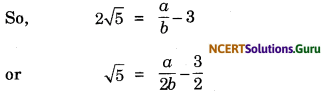Since, a and b are integers, therefore
$$\frac {a}{2b}$$ – $$\frac {3}{2}$$ is a rational number.
∴ √5 is a rational number.
But √5 is an irrational number.
This shows that our assumption is wrong.
So, 3 + 2 √5 is an irrational number.
Hence proved.
Example 4. Prove that √3 is irrational.
Solution:
If possible, let √3 be rational and let its simplest form be $$\frac{a}{b}$$
Then, a and b are integers having no common factor other than 1, and b ≠ 0.[on squaring both sides]
⇒ 3b2 = a2 …(i)
⇒ 3 divides a2 [ ∵ 3 divides 3b2]
⇒ 3 divides a [∵ 3 is prime and divides a2 ⇒ 3 divides a]
Let a = 3c for some integer c.
Putting a = 3c in (i), we get
3b2 = 9c2
⇒ b2 = 3c2
⇒ 3 divides b2 [ ∵ 3 divides 3c2]
⇒ 3 divides b [ ∵ 3 is prime and 3 divides b2 ⇒ 3 divides b]
Thus, 3 is a common factor of a and b. But, this contradicts the fact that a and b have no common factor other than 1.
The contradiction arises by assuming that √3 is rational.
Hence, √3 is irrational.Question 3.
The fo11owing rea1 numbers have decimal expansions as given be1ow. In each case, decide whether they are rational or not. If they are rational and of the form $$\frac{p}{q}$$, what can you say about the prime factors of q?
(i) 43.123456789
(ii) 0.120120012000120000….
(iii) $$43 . \overline{123456789}$$
Solution:
(i) 43.123456789 is terminating. So, it represents a rational number.
Thus,Thus, q = 109
(ii) 0.120120012000120000…. is non-terminating and non-repeating. So, it is an irrational number.
(iii) $$43 . \overline{123456789}$$ is non-terminating but repeating. So it is a rational.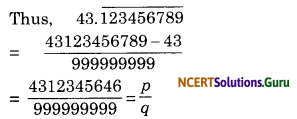Thus q = 999999999error: Content is protected !!